## Economic Operation of Power Plants Interview Questions and Answers:

1. Is the cost of fuel directly proportional to power output ?

Ans. No, the cost of fuel does not vary directly in proportion to the power output. It is directly proportional to the power output plus the losses in the conversion process.

2. What is input-output curve ?

Ans. The graphical relation between the fuel input, in Rs per hour, (or in million kilocalories per hour) and power output in MW for any power plant is known as input-output curve. A typical input-output curve is shown in Fig. 1.The Pmin is the minimum loading limit below which it is uneconomical or technically infeasible to operate the unit or plant and Pmax is the maximum output limit owing to its rating.

3. What is heat rate ?

Ans. Heat rate is defined as the ratio of input of fuel to corresponding output of power. It is found from the curve drawn by taking power output in MW on x-axis and fuel input in kilocalories per hour on y-axis. It is an indication of efficiency of the power plant.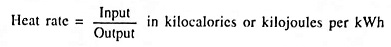4. What is heat rate curve ?

Ans. Heat rate curve is a graphical relation between the heat rate in kilojoules or kilocalories per MWh and power output in MW.

5. What is incremental rate of a machine ?

Ans. The incremental rate of a machine at any given output is the rate of change of the input with respect to the out­put i.e., the ratio of small change in input to a small change in output is known as incremental rate of a ma­chine. Numerically it is equal to the slope of the input/output curve at a point corresponding to that output. Mathematically, it is the first derivative of the input with respect to the output.

6. What is incremental cost ?

Ans. In the input-output characteristic if input is expressed in kJ/hour and output in MW, the slope of the curve gives incremental fuel rate in kJ/MWh and if the input is ex­pressed in Rs per hour, the slope of the curve gives incre­mental fuel cost in Rs/MWh. Mostly incremental fuel cost is taken as the incremental cost (IC) and forms the basis of load division.

7. How is incremental cost calculated ?

Ans. In the input-output characteristic if the input is expressed in Rs/hour and output in MW, the slope of the curve gives incremental fuel cost in Rs/MWh. Mostly incremental fuel cost is taken as the incremental cost.

i.e., incremental cost is the ratio of change in input to cor­responding change in output power.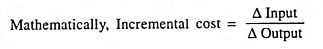8. What are the units of incremental cost ?

Ans. The units of incremental cost are Rs per MWhr.

9. What does economic load scheduling mean ?

Ans. By economic load scheduling, we mean to determine the generations of different power plants such that the total operating cost is minimum and at the same time the total demand and losses at any instant is met by the total gen­eration.

10. Why is incremental fuel cost generally taken as total in­cremental cost for the purpose of load allocation to ther­mal plants ?

Ans. The total incremental cost is made up of incremental fuel cost plus incremental cost of other items, such as water, supplies, wages etc. It is very difficult to express costs of water, supplies, wages etc, directly as a function of output. Therefore, for simplification, these costs are taken as fixed percentage of incremental fuel cost. Hence, the incremen­tal fuel cost is usually taken as total incremental cost.

11. What is Lagrangian multiplier ?

Ans. Lagrangian multiplier, denoted by Greek letter λ, is the incremental cost of the unit in Rs per MWh. For optimum economy every generating unit should he operated at the same incremental cost, λ.

12. Why should transmission losses be included in incremen­tal cost criterion ?

Ans. When a generating unit supplies power over a transmission line, and when generation of the unit increases, the same increment is not delivered to the load. The change in power at the load end or bus is the original incremental less the incremental loss in the line. Thus, the incremental loss in the transmission line is the function of power of the gen­erating unit (or plant) and the line characteristic. Evidently the incremental cost curve at the generating unit or plant is to be modified to include the cost of the incremental losses in the line.

13. Write transmission loss B-matrix.

Ans. The transmission losses, PL can be computed utilizing loss formula coefficients or “B-coefficients”. These B-coeffi­cients for a given system are assumed to remain constant and their evaluation depends upon the open-circuit imped­ance matrix of the system network.

The transmission losses are given as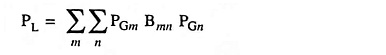where PL are transmission losses, PG is plant loading, B are loss coefficients, all in per units (pu) and ∑m and ∑n indicate independent summation to include all sources

or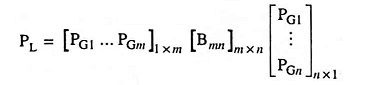The B-coefficient matrix is written as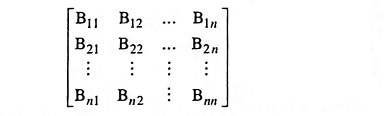where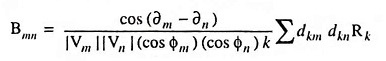and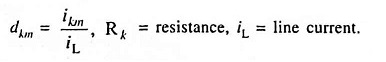14. What are coordination equations ?

Ans. Coordination equations represent the coordination between various generating units as regards to their load allocation. These equations provide relationships between cost of en­ergy on one hand and power output on the other.

15. Define penalty factor.

Ans. Penalty factor is defined as the ratio of small change in power of one unit to the small change in power received when only this unit supplies small change in power re­ceived.

16. What do you mean by iterative procedure ?

Ans. In an iterative procedure, the coordination equations are solved by method of successive approximations. A first guess of initial solution is assumed and error from actual value is determined. The assumed value is corrected again and again so as to reduce error to acceptable level.

Scroll to Top